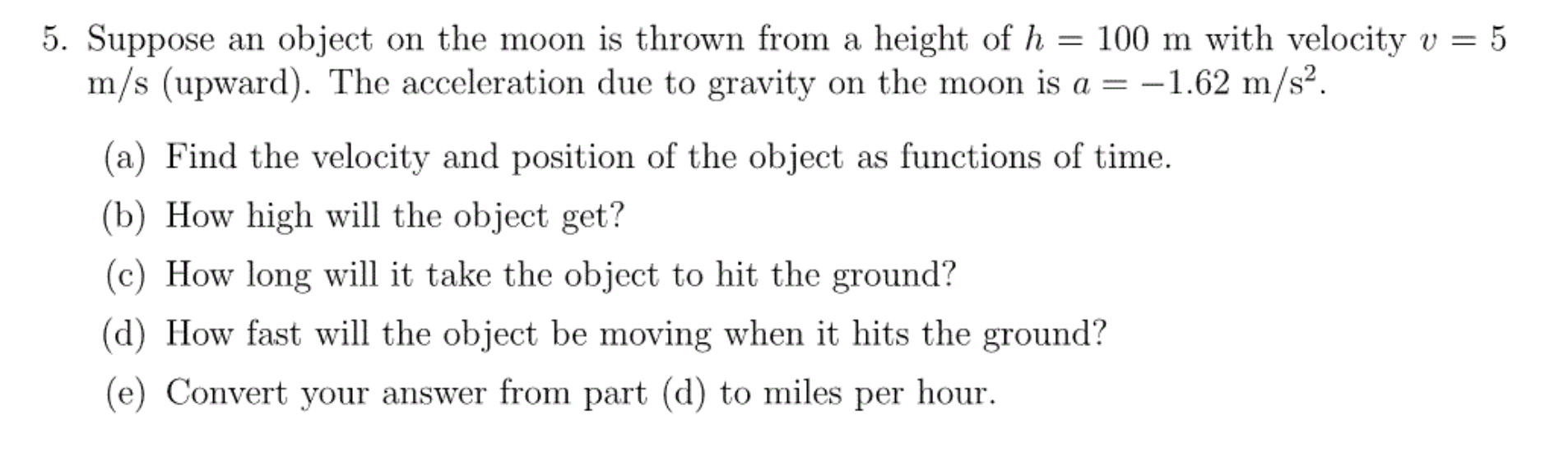# 5. Suppose an object on the moon is thrown from a height of h = 100 m with velocity vm/s (upward). The acceleration due to gravity on the moon is a = -1.62 m/s2.5(a) Find the velocity and position of the object as functions of time(b) How high will the object get?(c) How long will it take the object to hit the ground?(d) How fast will the object be moving when it hits the ground?(e) Convert your answer from part (d) to miles per hour

Question
2 viewshelp_outlineImage Transcriptionclose5. Suppose an object on the moon is thrown from a height of h = 100 m with velocity v m/s (upward). The acceleration due to gravity on the moon is a = -1.62 m/s2. 5 (a) Find the velocity and position of the object as functions of time (b) How high will the object get? (c) How long will it take the object to hit the ground? (d) How fast will the object be moving when it hits the ground? (e) Convert your answer from part (d) to miles per hour fullscreen
check_circle

Step 1

Since we only answer up to 3 sub-parts, we’ll answer the first 3. Please resubmit the question and specify the other subparts (up to 3) you’d like answered.

v0= initial velocity = 5 m/s

h0=initial height = 100 m

v(t)= velocity at time t.

h(t)= height at time t.

v(t) = v0 + at, in this we plug the known values.

Answer(a): Velocity of the object at time t = v(t)= 5-1.62t

Step 2

Position at time t= h(t)= h0 + v0t + (1/2)at^2

Answer(a): Position at time t= h(t) = 100+5t - 0.81t^2

Step 3

b) At the maximum height velocity will be 0. so we set v(t)=0 and solve for...

### Want to see the full answer?

See Solution

#### Want to see this answer and more?

Solutions are written by subject experts who are available 24/7. Questions are typically answered within 1 hour.*

See Solution
*Response times may vary by subject and question.
Tagged in

### Calculus